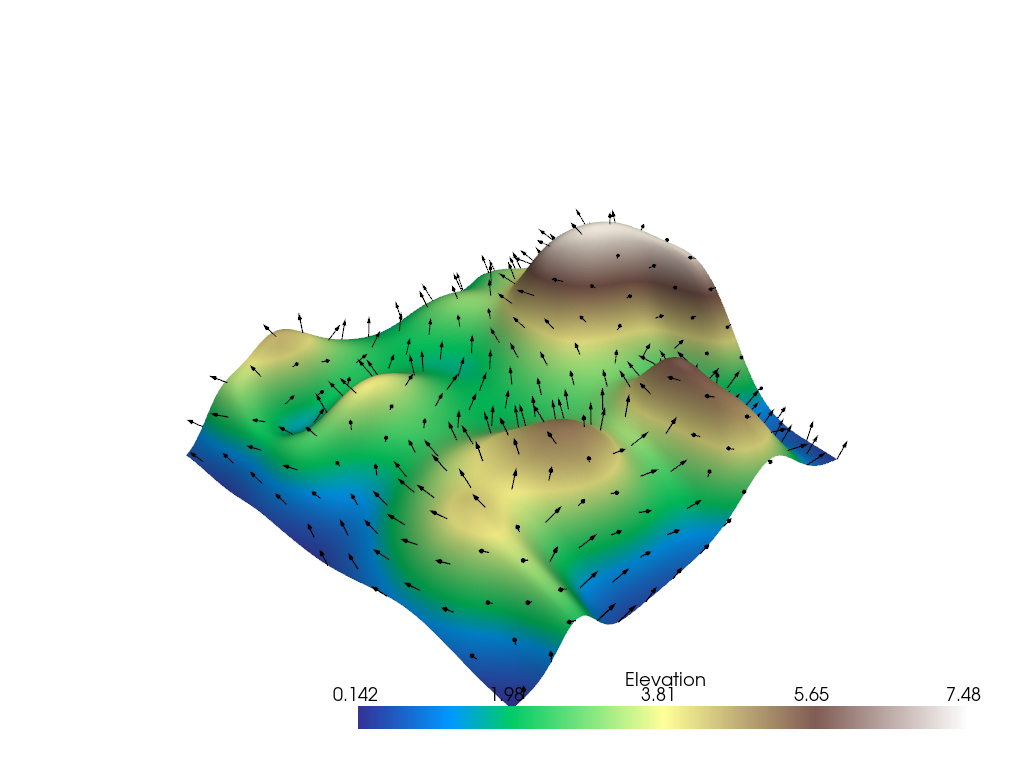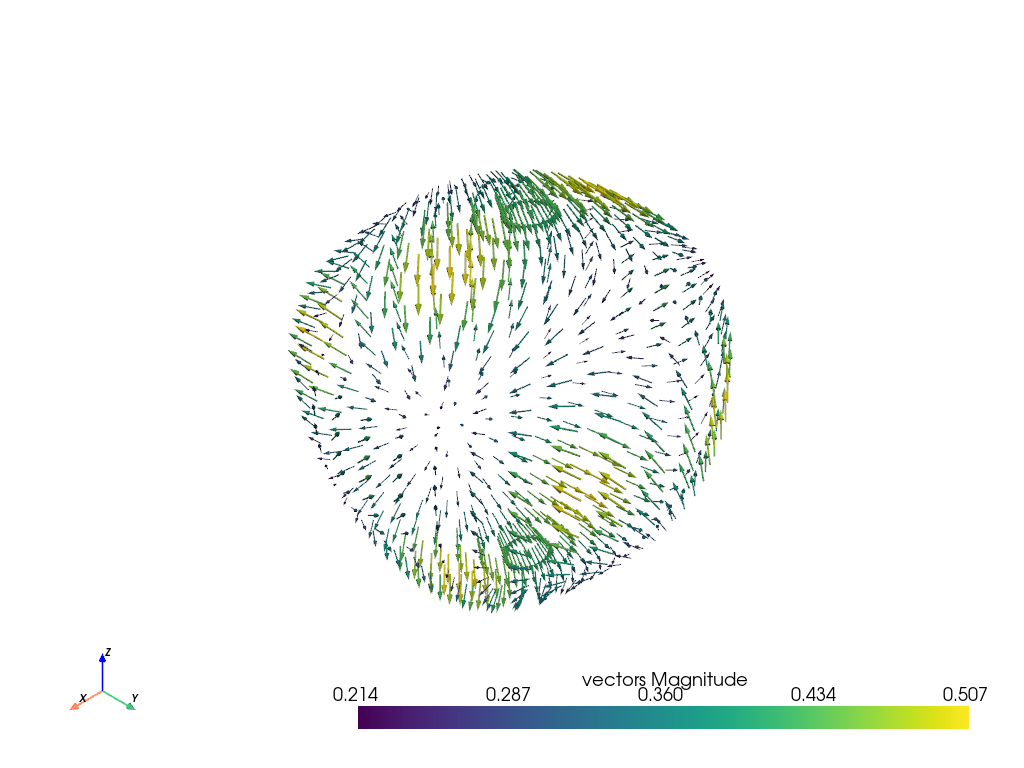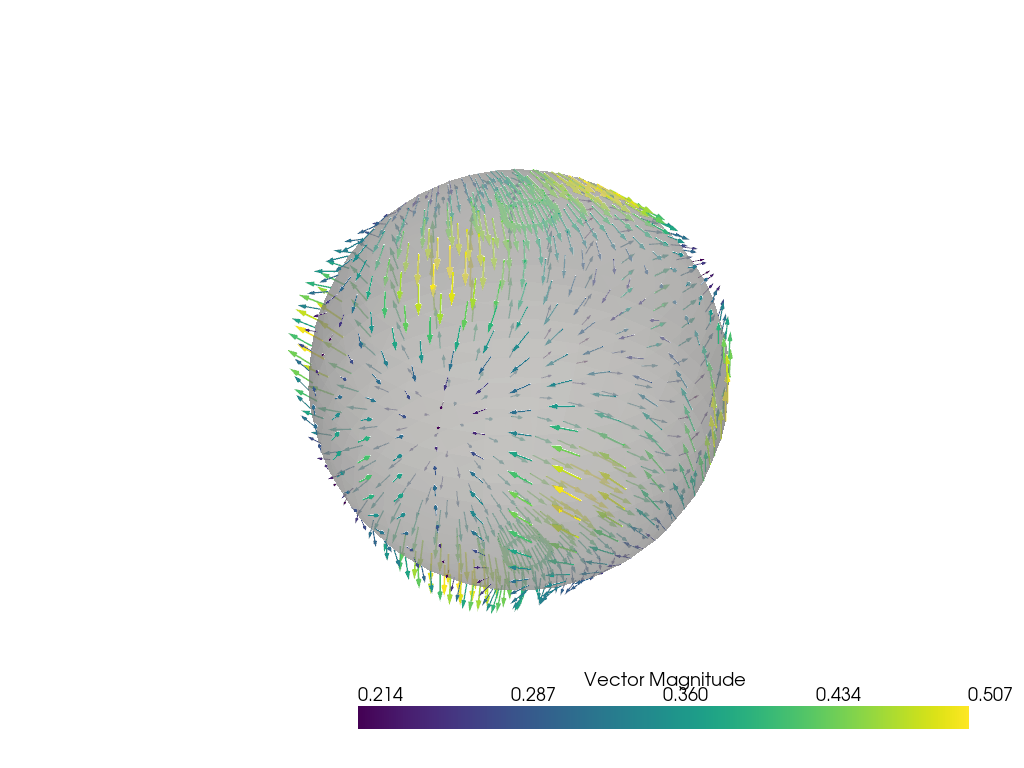# Plotting Glyphs (Vectors or PolyData)#

Use vectors in a dataset to plot and orient glyphs/geometric objects.

```import numpy as np
import pyvista as pv
from pyvista import examples
```

Example dataset with normals

```mesh = examples.load_random_hills()
```

Glyphying can be done via the `pyvista.DataSetFilters.glyph()` filter

```help(mesh.glyph)
```
```Help on method glyph in module pyvista.core.filters.data_set:

glyph(orient=True, scale=True, factor=1.0, geom=None, indices=None, tolerance=None, absolute=False, clamping=False, rng=None, progress_bar=False) method of pyvista.core.pointset.PolyData instance
Copy a geometric representation (called a glyph) to the input dataset.

The glyph may be oriented along the input vectors, and it may
be scaled according to scalar data or vector
magnitude. Passing a table of glyphs to choose from based on
scalars or vector magnitudes is also supported.  The arrays
used for ``orient`` and ``scale`` must be either both point data
or both cell data.

Parameters
----------
orient : bool or str, optional
If ``True``, use the active vectors array to orient the glyphs.
If string, the vector array to use to orient the glyphs.
If ``False``, the glyphs will not be orientated.

scale : bool, str or sequence, optional
If ``True``, use the active scalars to scale the glyphs.
If string, the scalar array to use to scale the glyphs.
If ``False``, the glyphs will not be scaled.

factor : float, optional
Scale factor applied to scaling array.

geom : vtk.vtkDataSet or tuple(vtk.vtkDataSet), optional
The geometry to use for the glyph. If missing, an arrow glyph
is used. If a sequence, the datasets inside define a table of
geometries to choose from based on scalars or vectors. In this
case a sequence of numbers of the same length must be passed as
``indices``. The values of the range (see ``rng``) affect lookup
in the table.

indices : tuple(float), optional
Specifies the index of each glyph in the table for lookup in case
``geom`` is a sequence. If given, must be the same length as
``geom``. If missing, a default value of ``range(len(geom))`` is
used. Indices are interpreted in terms of the scalar range
(see ``rng``). Ignored if ``geom`` has length 1.

tolerance : float, optional
Specify tolerance in terms of fraction of bounding box length.
Float value is between 0 and 1. Default is None. If ``absolute``
is ``True`` then the tolerance can be an absolute distance.
If ``None``, points merging as a preprocessing step is disabled.

absolute : bool, optional
Control if ``tolerance`` is an absolute distance or a fraction.

clamping : bool, optional
Turn on/off clamping of "scalar" values to range. Default ``False``.

rng : tuple(float), optional
Set the range of values to be considered by the filter
when scalars values are provided.

progress_bar : bool, optional
Display a progress bar to indicate progress.

Returns
-------
pyvista.PolyData
Glyphs at either the cell centers or points.

Examples
--------
Create arrow glyphs oriented by vectors and scaled by scalars.
Factor parameter is used to reduce the size of the arrows.

>>> import pyvista
>>> from pyvista import examples
>>> arrows = mesh.glyph(scale="Normals", orient="Normals", tolerance=0.05)
>>> pl = pyvista.Plotter()
>>> actor = pl.add_mesh(mesh, scalars="Elevation", cmap="terrain",
...                     show_scalar_bar=False)
>>> pl.show()

See :ref:`glyph_example` and :ref:`glyph_table_example` for more
examples using this filter.
```

Sometimes you might not want glyphs for every node in the input dataset. In this case, you can choose to build glyphs for a subset of the input dataset by using a merging tolerance. Here we specify a merging tolerance of five percent which equates to five percent of the bounding box’s length.

create a subset of arrows using the glyph filter

```arrows = mesh.glyph(scale="Normals", orient="Normals", tolerance=0.05)
```
```p = pv.Plotter()
p.show()
```A common approach is to load vectors directly to the mesh object and then access the `pyvista.DataSet.arrows` property to produce glyphs.

```sphere = pv.Sphere(radius=3.14)

# make cool swirly pattern
vectors = np.vstack(
(
np.sin(sphere.points[:, 0]),
np.cos(sphere.points[:, 1]),
np.cos(sphere.points[:, 2]),
)
).T
vectors
```
```array([[-3.4861004e-16,  1.0000000e+00, -9.9999875e-01],
[ 3.4861004e-16,  1.0000000e+00, -9.9999875e-01],
[-3.3300975e-01,  1.0000000e+00, -9.9980003e-01],
...,
[-8.3088565e-01,  9.7835207e-01, -9.8625994e-01],
[-6.1331964e-01,  9.9016821e-01, -9.9718851e-01],
[-3.2600534e-01,  9.9750996e-01, -9.9980003e-01]], dtype=float32)
```
```# add and scale
sphere["vectors"] = vectors * 0.3
sphere.set_active_vectors("vectors")

# plot just the arrows
sphere.arrows.plot()
```Plot the arrows and the sphere.

```p = pv.Plotter()